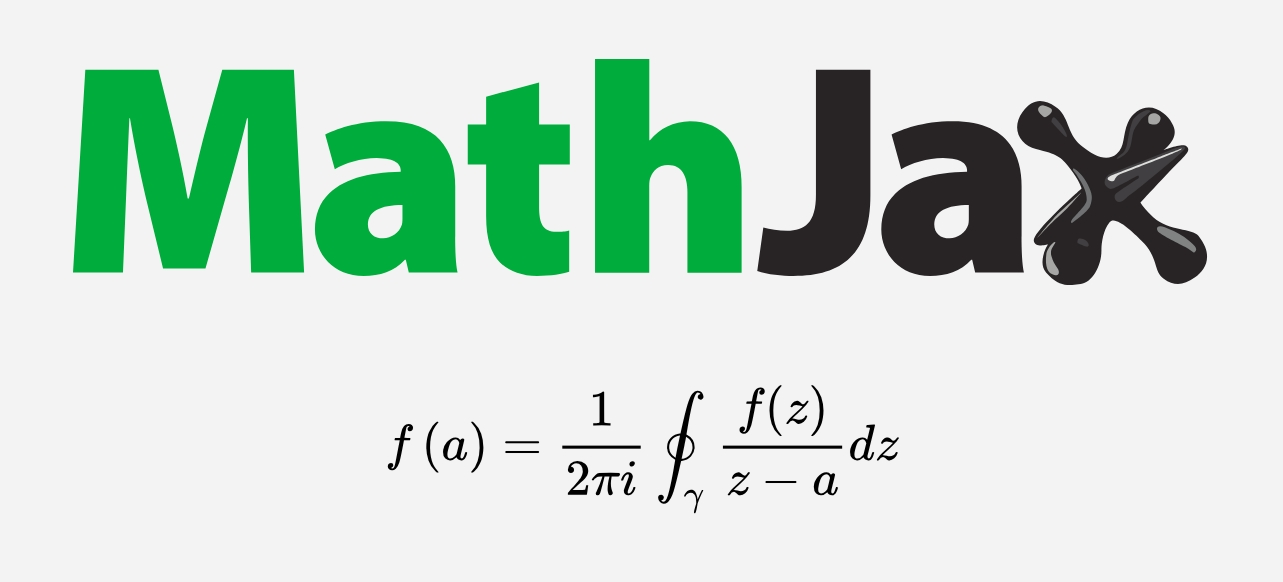# Hexo 中 MathJax 的静态显示（svg）1. 动态加载，渲染还要时间；
2. 有个右键菜单，感觉没必要。

## #操作步骤

1）安装

~/blog $npm i -g gulp@3.9.1 ~/blog$ npm i -S gulp@3.9.1 gulp-mathjax-page


2）新建

// 文件位置：~/blog/gulpfile.js

var gulp = require('gulp')
var mathjax = require('gulp-mathjax-page')

gulp.src('./public/**/*.html')
.pipe(mathjax({
mjpageConfig: {
format: ['TeX'],
singleDollars: true,
cssInline: false,
mhchem: {legacy: true}
},
mjnodeConfig: {
svg: true,
css: false,
speakText: false
}
}))
.pipe(gulp.dest('./public'))
})


3）样式

/* 文件位置：~/blog/themes/next/source/css/_custom/custom.styl */

.mjpage__block {
display: inline-block;
text-align: center;
width: 100%;
overflow-x: auto;
vertical-align: bottom;
}

/* 如果出现尺寸过大的问题，则加上以下代码 */
.mjpage {
font-size: 10px;
}


4）使用

~/blog $hexo clean && hexo g && gulp mathjax && hexo d  本地无法在浏览器直接 hexo s 查看预览，但可以 gulp mathjax 后去 public 文件夹下找到相应文件并用浏览器打开预览。当然，这样预览过于麻烦了，所以建议直接用 Mathjax 官网的 Live Demo 预览，部署前再用上面这种方法确认一遍即可。 ## #效果展示 注意：我已从 Hexo 迁移到 Hugo，下面的公式是由$\KaTeX$在线渲染的，而非服务端预先渲染好的 SVG。 爱因斯坦场方程： $$G_{μν} = R_{μν} - \frac{1}{2}g_{μν}R = -κT_{μν}$$ 克莱因—戈登（Klein-Gordon）方程，描述玻色子： $$-h^2\frac{\partial^2}{\partial{t}^2}\psi(r,t) = [-h^2c^2\frac{\partial^2}{\partial{r}^2}+m^2c^4]\psi(r,t)$$ 上面方程是时间和空间的二阶导数，不简洁，于是狄拉克将之化为时间和空间的一阶导数，狄拉克（Dirac）方程，描述费米子： $$i\hbar\frac{\partial}{\partial{t}}\psi(r,t) = [-i\hbar{c}\alpha\cdot\frac{\partial}{\partial{r}}+mc^2\beta]\psi(r,t)$$ 物理学的大前提：自然规律应该是简洁的！ 麦克斯韦方程组： $$\begin{cases} \nabla\times\mathbf{E} &= -\frac{\partial\mathbf B}{\partial t} \\ \nabla\times\mathbf{B} &= \mu_0\mathbf{J} + \mu_0\epsilon_0\frac{\partial\mathbf E}{\partial t} \\ \nabla\cdot\mathbf{E} &= \frac{\rho}{\epsilon_0} \\ \nabla\cdot\mathbf{B} &= 0 \end{cases}$$ 四个独立方程按上面顺序分别是：法拉第电磁感应定律、毕奥沙伐尔定律、库仑定律、磁场无源场。 麦克斯韦为了解释电容器可以通交流这个现象，在毕奥沙伐尔定律后面加上$\mu_0\epsilon_0\frac{\partial\mathbf E}{\partial t}$（位移电流假说），于是将本来独立的四个方程紧紧联系在一起，构成一个方程组。 用微积分解微分方程，得到两个波动方程： $$\frac{\partial^{2}\mathbf E_x}{\partial\mathbf Z^{2}} - \partial\partial_0\mu\mu_0\frac{\partial^{2}\mathbf E_x}{\partial t^{2}} = 0$$ $$\frac{\partial^{2}\mathbf H_y}{\partial\mathbf Z^{2}} - \partial\partial_0\mu\mu_0\frac{\partial^{2}\mathbf H_y}{\partial t^{2}} = 0$$ 上面第一个方程是电场的，第二个是磁场的。 牛顿的时候波动方程的结论： 1. 只要这个物理量满足波动方程，那么这个物理量就肯定以波的形式向外传播。 2. 对时间二阶导数前面的常数（$\partial\partial_0\mu\mu_0\$）开根号再导数，就是这个波的传播速度。

$$ν = \frac{1}{\sqrt{\partial\partial_0\mu\mu_0}} \approx 300000 (km/s)$$

1. 电磁以波动形式传播
2. 光是一种电磁波（光速当时已测出）

## #化学方程

$$\ce{SO4^2- + Ba^2+ -> BaSO4 v}$$

$$n + \ce{ ^{235}_{92} U -> ^{144}_{56} Ba + ^{89}_{36} Kr } + 3n + 200\;Mev$$

\begin{cases} \begin{aligned} &&\ce{ ^{1} H + ^{1} H & -> ^{2} D } + e^{+} + ν \\ \text{then}\;&&\ce{ ^{2} D + ^{1} He & -> ^{3} He } + \gamma \\ \text{then}\;&&\ce{ ^{3} He + ^{3} He & -> ^{4} He + ^{1} H + ^{1} H } \end{aligned} \end{cases}

## #提示说明

\left\{
\begin{array}...\end{array}
\right.

Enjoy it! ☕